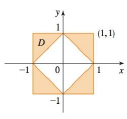Chapter 15.2, Problem 57E

Chapter
Section
Textbook Problem

Express D as a union of regions of type I or type II and evaluate the integral.57. ∬ D x 2   d ATo determine

To express: The region D as a union of regions of type 1 or type 2 and find the value of given double integral.

Explanation

Region of type 1:

A plane region D is said to be of type 1 if it lies between two continuous functions of x.

That is, D={(x,y)|axb,g1(x)yg2(x)} ,where g1(x) and g2(x) are the continuous functions of x.

Region of type 2:

A plane region D is said to be of type 2 if it lies between two continuous functions of y.

That is, D={(x,y)|ayb,h1(y)xh2(y)} ,where h1(y) and h2(y) are the continuous functions of y.

Calculation:

Since the given region D is symmetric about both x and y axes, it is enough to find the value of integral under D in the first octant only and multiply it with 4. In the first octant, consider the region D is of type 1, x varies from 1y to 1 and y varies from 0 to 1. So, the value of the double integral is,

Df(x,y)dA=4011y1x2dxdy

First, compute the integral with respect to x.

011y1x2dxdy=01[1y1x2dx]dy=01[x33]1y1dy

Apply the limit value for x,

01

Still sussing out bartleby?

Check out a sample textbook solution.

See a sample solution

The Solution to Your Study Problems

Bartleby provides explanations to thousands of textbook problems written by our experts, many with advanced degrees!

Get Started

If f(x) = (x a)(x b)(x c), show that f(x)f(x)=1xa+1xb+1xc

Single Variable Calculus: Early Transcendentals, Volume I

In Problems 1-8, find the derivative of each function.

Mathematical Applications for the Management, Life, and Social Sciences

For f(x) = 5 + g(x), f(x) = _____. a) 5g(x) b) 5 + g(x) c) 0 g(x) d) g(x)

Study Guide for Stewart's Single Variable Calculus: Early Transcendentals, 8th

True or False: is a convergent series.

Study Guide for Stewart's Multivariable Calculus, 8th